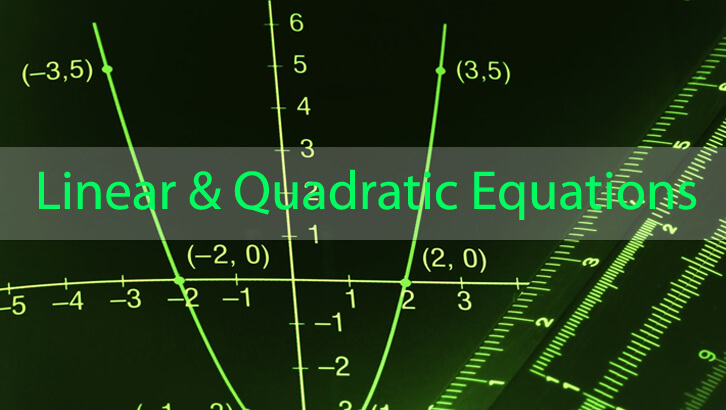# CAT Linear Equations

Question
A system of equations has 3 equations
3x + 4y + 5z = 11, 4x + 5y + 3z = 14 and 2x + 3y + kz = 6.
If the system of equations has no solution, find k.

Explanation
This is a very interesting question. If we can generate one of the equations from the other two, we can then say that the system has infinite solutions. If we can generate one of the equations from the other two, but with the constant part alone being different, this would be akin to having parallel lines when we are dealing with 2 variables and that would result in the system having no solutions. So, lets look for that.

So, we need to find some way where a(3x + 4y + 5z) + b(4x + 5y + 3z) =  2x + 3y + kz.
We need to find k. In other words, we need to find a, b such that a(3x + 4y) + b(4x + 5y ) =  2x + 3y . Then said a, b would give us k.

Or, we are effectively solving for
3a + 4b = 2
4a + 5b = 3

Subtracting one from the other, we get a + b = 1. 3a + 3b = 3. Or, b = -1. a = 2.

Now, k = 5a + 3b = 10 -3 = 7.

If k = 7, this system of equations would result in no solutions.

If k were 7, the system of equations should be 3x + 4y + 5z = 11, 4x + 5y + 3z = 14 and 2x + 3y + 7z = 6.
First equation * 2 – second equation would give us the equation 2x + 3y + 7z = 8.

Now, 2x + 3y + 7z cannot be 6 and 8 at the same time. So, this system of equations has no solution.

Yeah, the more mechanical, rather deceptively straight-forward-looking determinant method is also there. But where is the joy in that.